# Rotate a MapControl content to show a polyline horizontal

1014
5
07-17-2020 07:08 AM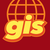New Contributor III

Hi,

How can I rotate a MapControl content to show a polyline data always horizontal irrespective of its angle. I am using a Projected Coordinate System Map. I tried binding the buffered extent of the polyline and setting the camera heading to the line segment angle converted to degrees. It doesn't work as expected.

``````        private async Task SetMapContent()
{
var mapView = MapView.Active;
double Rad2Degrees = 180 / Math.PI;
if (mapView != null && lineGeom != null)
{
var segment = await QueuedTask.Run(() =>
{
var lb = new LineBuilder(lineGeom.Points.FirstOrDefault(), lineGeom.Points.LastOrDefault());
return lb.ToSegment();
});

var bearing = segment.Angle * Rad2Degrees;
Camera camera = mapView.Camera;

ViewContent = MapControlContentFactory.Create(MapView.Active.Map, camera, MapView.Active.Map.DefaultViewingMode);
}
}‍‍‍‍‍‍‍‍‍‍‍‍‍‍‍‍‍‍‍‍‍‍‍‍‍‍‍‍‍‍‍‍‍‍‍‍‍‍‍‍‍‍‍‍‍‍‍‍‍‍‍‍‍‍‍‍‍``````

and then below code to set the extent everytime the line data changes

``````await QueuedTask.Run(() =>
{
var bufferPoly = GeometryEngine.Instance.Buffer(lineGeom, 0.1);
MapPoint cent = GeometryEngine.Instance.Centroid(lineGeom);
var ext = EnvelopeBuilder.CreateEnvelope(bufferPoly.Extent);

if (ext != null)
{
System.Windows.Application.Current.Dispatcher.Invoke(async () =>
{
ViewContentExtent = ext;
NotifyPropertyChanged(() => ViewContentExtent);
});
}
});‍‍‍‍‍‍‍‍‍‍‍‍‍‍‍‍‍‍‍‍‍‍‍‍‍‍‍‍‍‍‍‍‍‍‍‍‍‍‍‍‍‍‍‍‍‍‍‍‍‍‍‍‍‍‍‍‍‍‍‍‍‍‍``````

Any suggestions to get this working ?

Thanks,

Sai

Tags (1)
1 Solution

Accepted Solutions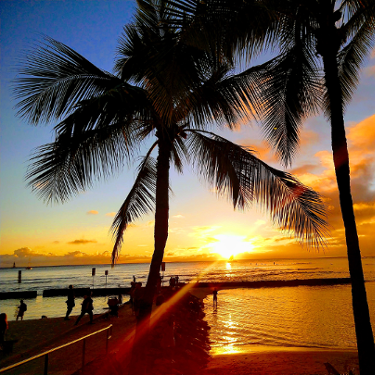byEsri Regular Contributor

Hi Sai,

The solution is a bit complicated but in essence you need to set the mapcontrol's map extent as follows.  This approach requires that the MapControl's aspect ratio is fixed:

1. Create a rectangle with your given line as the centerline, with the rectangle having the MapControl's aspect ratio.
2. Now you need to compute another rectangle that is centered around the given line's center point and this rectangle cannot intersect the rectangle from step 1 (in essence the step 2 rectangle has to fit inside the rectangle from step 1).  To compute this rectangle I am using the step 1 rectangle's extent in order to intersect either diagonal with the step 1 rectangle.
3. Set the MapControl's extent using the extent of the step 2 rectangle.

In the screenshot below you can see the rectangle created in step 1 with a cyan colored outline, the rectangle in step 2 is marked by the two red corner point markers.  The cyan rectangle is also displayed in the overlay window.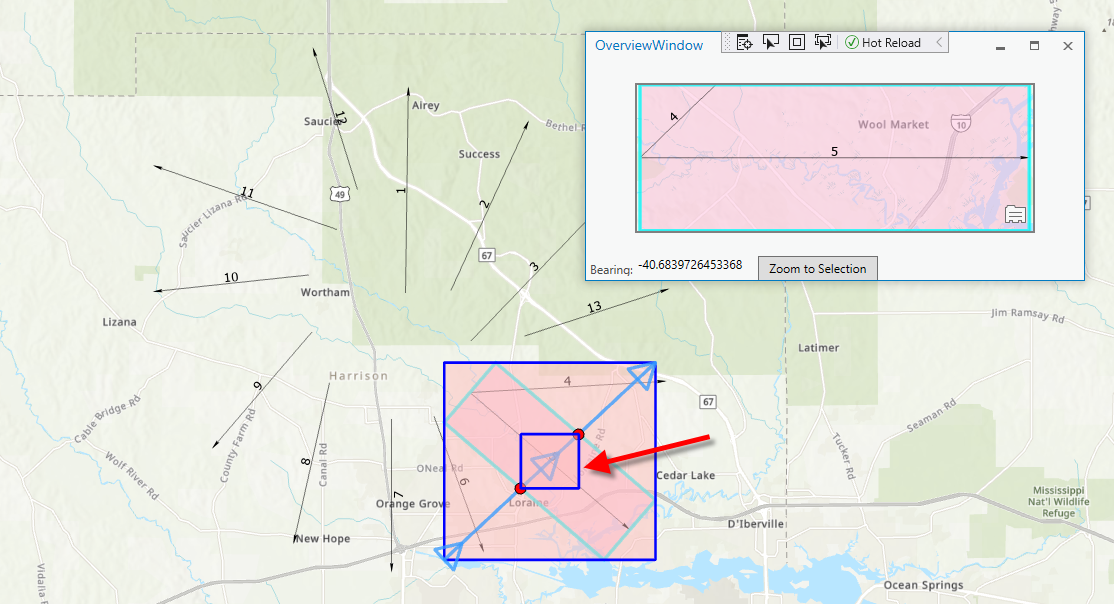I attached my code and data.  In order to build the code you have to first use the 'Pro Fix References' tool.  When running the add-in you have to first use the map control tool to click the desired line (to be displayed horizontally in the overview window) and then click the "Step 1" button which executes the algorithm explained above.  Please use the attached 'Lines.zip' project file because it contains both sample lines and a polygon layer used for debug purposes.

5 RepliesbyEsri Regular Contributor

It seem to work for me.  Just to summarize:

When you set the camera's heading the following (from API help) applies for the heading property:

Gets or sets the Heading of the Camera, in degrees from North. 0 is North, 90 is West, 180 is South, -90 is East, etc. This property applies to both 2D and 3D views.

When you compute the angle for you line the following applies:Note that start and end point (i.e. the direction of the line) are important in the computation, but you get values of 0 through 180 if your angle is 'above' the 0 degree horizon (see image above) and 0 through -180 if your angle is below the horizon.   i made a sample and it appears to work fine for me.  Below i sketched a 90 degree line - which points my heading 'West' ... so i think that's correct: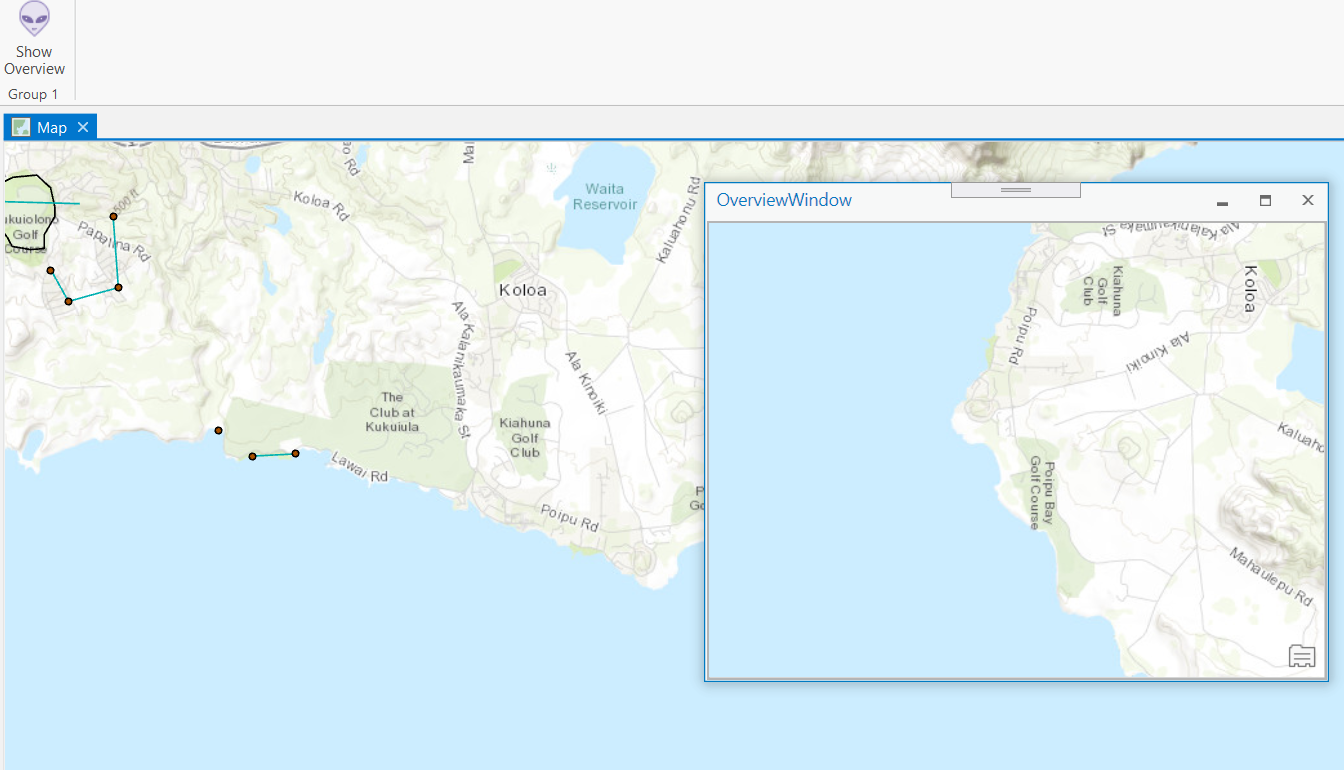So can you describe what doesn't work as expected and also what coordinate system you're using?New Contributor III

Thanks for looking into this Wolfgang Kaiser‌.

I was thinking the issue I was looking at is caused by rotation, when I put up a sample to share with you I figured out the issue was different.

I still am sharing the sample to get some help with zooming to correct extent for few of the lines highlighted below. Other lines zoom to exact extent whereas the highlighted ones does not. Please check and let me know what can I do here.Attached sample code and test data.

Regards,
SaibyEsri Regular Contributor

Hi Sai,

It turns out that the problem is caused by the aspect ratio of the MapControl's display area.  Because you are using the 'extent' of the line (and its envelope) as the new extent for the MapControl it is important to ensure that the MapControl's aspect ratio supports both the new 'height' and 'width' without having to make adjustments to the width.  in your case the MapControl was wider than tall and hence the 'edge cases' you listed above, where the line's extent area nearly becomes as wide as it is tall, the MapControl had to 'widen' the extent in order to honor both x and y requirements of the newly specified extent.  You can see the arrows of the selected line are far from the map display border.

Here is an example - note that the boxes display the line's extent on the active map:You can see that the OverviewWindow's MapControl is wider than tall and the extent of the 'highlighted' line is nearly square ... consequently the MapControl has to extent the width in order to honor the full height extent.

A simple solution would be to make the MapControl square or taller than wide and now the same sample works: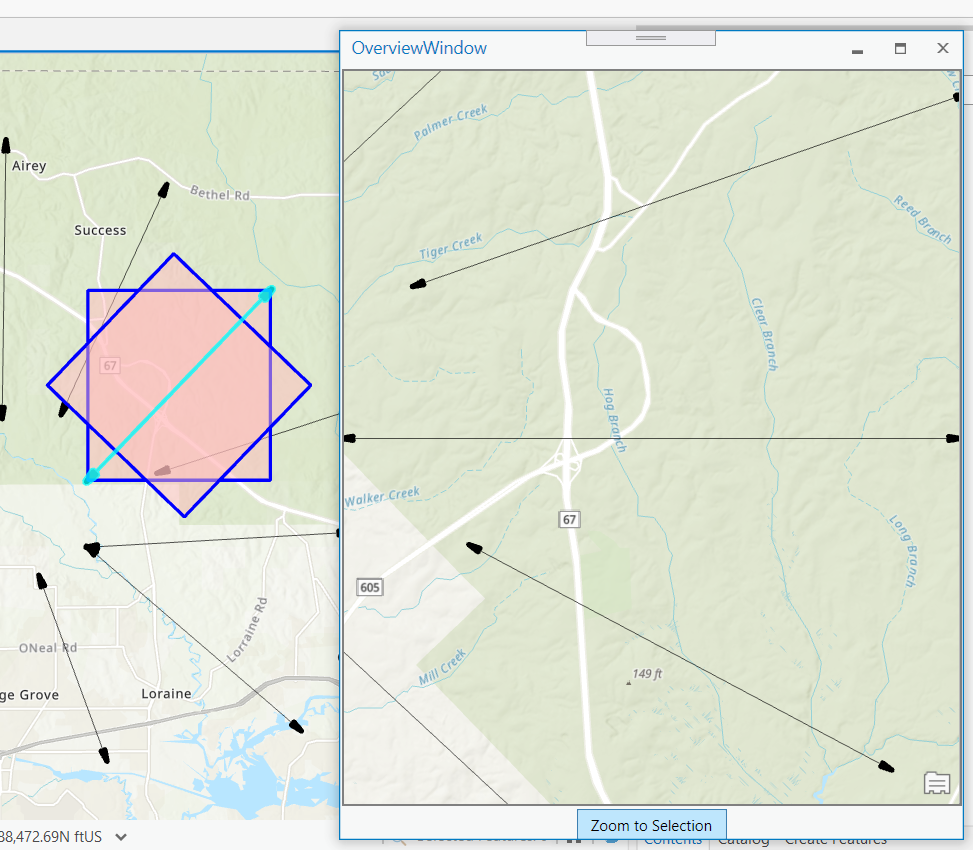byEsri Regular Contributor

Hi Sai,

The solution is a bit complicated but in essence you need to set the mapcontrol's map extent as follows.  This approach requires that the MapControl's aspect ratio is fixed:

1. Create a rectangle with your given line as the centerline, with the rectangle having the MapControl's aspect ratio.
2. Now you need to compute another rectangle that is centered around the given line's center point and this rectangle cannot intersect the rectangle from step 1 (in essence the step 2 rectangle has to fit inside the rectangle from step 1).  To compute this rectangle I am using the step 1 rectangle's extent in order to intersect either diagonal with the step 1 rectangle.
3. Set the MapControl's extent using the extent of the step 2 rectangle.

In the screenshot below you can see the rectangle created in step 1 with a cyan colored outline, the rectangle in step 2 is marked by the two red corner point markers.  The cyan rectangle is also displayed in the overlay window.I attached my code and data.  In order to build the code you have to first use the 'Pro Fix References' tool.  When running the add-in you have to first use the map control tool to click the desired line (to be displayed horizontally in the overview window) and then click the "Step 1" button which executes the algorithm explained above.  Please use the attached 'Lines.zip' project file because it contains both sample lines and a polygon layer used for debug purposes.New Contributor III

As you have mentioned, the solution is a bit complicated. But this is the closest solution to achieve what I want to do. A flag on MapControl to respect the aspect ratio or set the extent as is would be of much help.

Thanks Wolfgang Kaiser